# 1. 定义

y = a x 2 + b x + c ( a ≠ 0 ) y=ax^2+bx+c(a\neq0)

# 2. y=ax^2图象与性质

y = x 2 y=x^2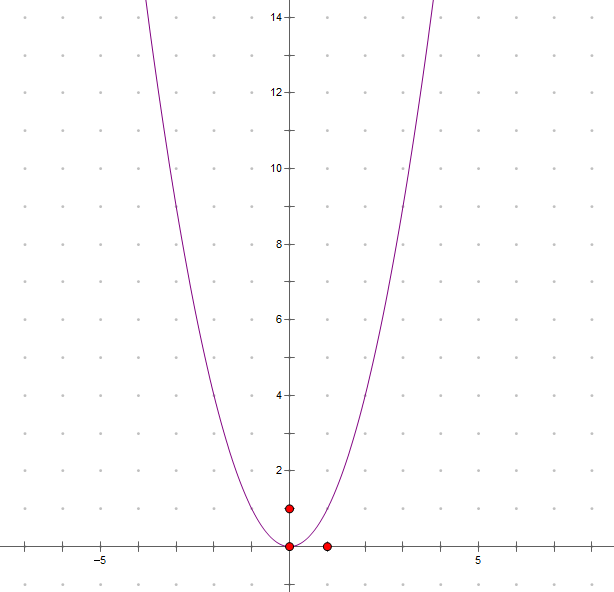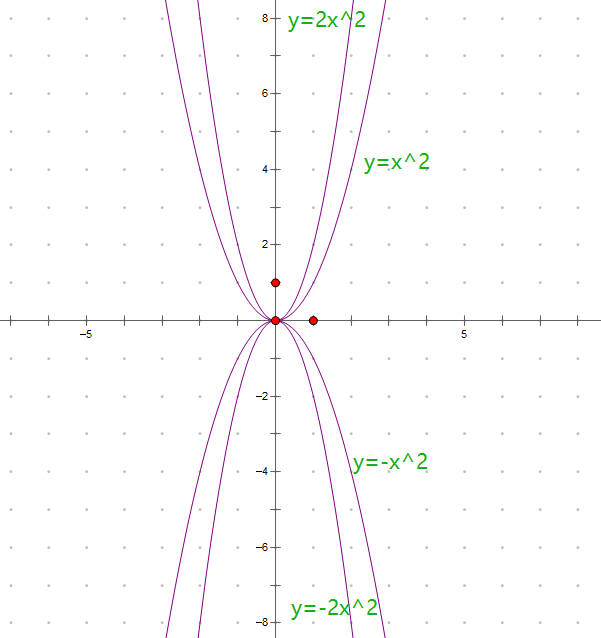y = a x 2 y=ax^2

• a>0时，抛物线开口向上，a越大，抛物线开口越小。这是因为a越大，增长的越快。
• a<0时，抛物线开口往下，a越小，抛物线开口越小。a为负值时，x越大，函数值就越小。

# 3. y=a(x-h)^2+k图象和性质

## 3.1 k值对图象的影响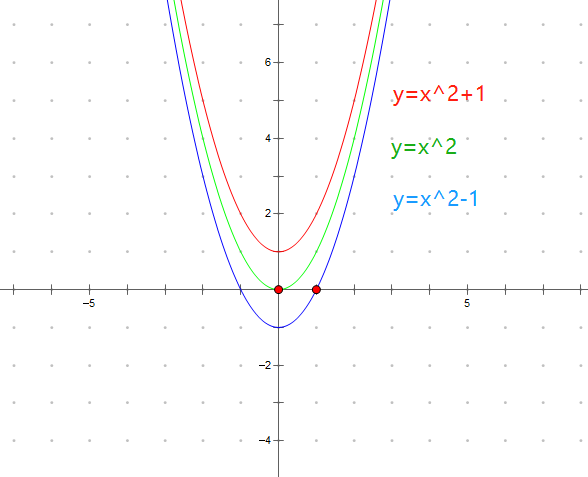## 3.2 h值对图象的影响

a ( x − h ) 2 + k a(x-h)^2+k
h变化对图象的影响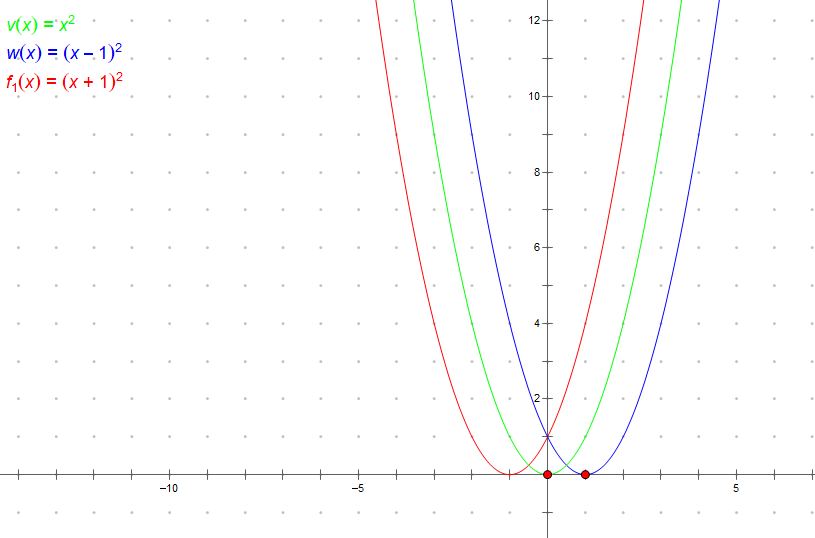## 3.3 a值对图象的影响

a ( x − h ) 2 + k a(x-h)^2+k
a变化对图象的影响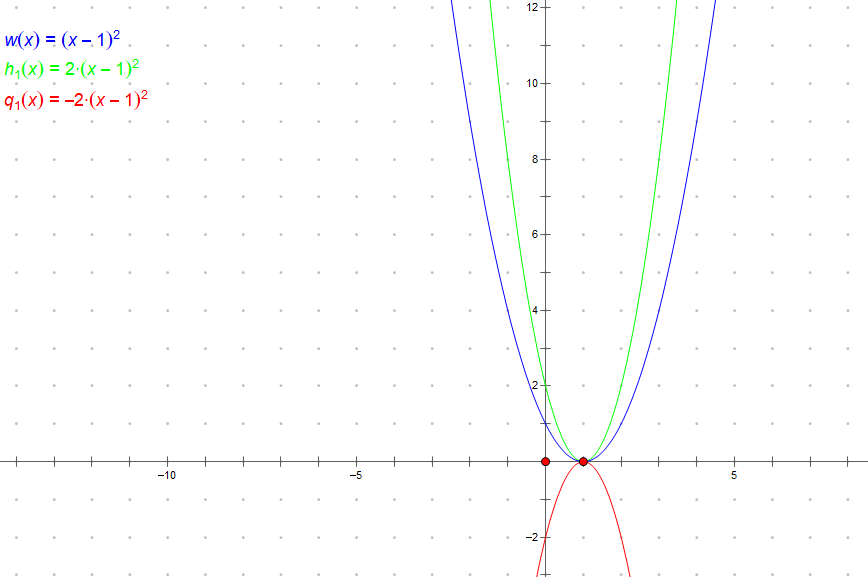# 4. y=ax^2+bx+c图象与性质

y = a ( x + b 2 a ) 2 + 4 a c − b 2 4 a y=a{(x+\frac b{2a})}^2+\frac{4ac-b^2}{4a}

x = − b 2 a x=-\frac b{2a}

( − b 2 a , 4 a c − b 2 4 a ) (-\frac b{2a},\frac{4ac-b^2}{4a})

# 5. 二次函数与一元二次方程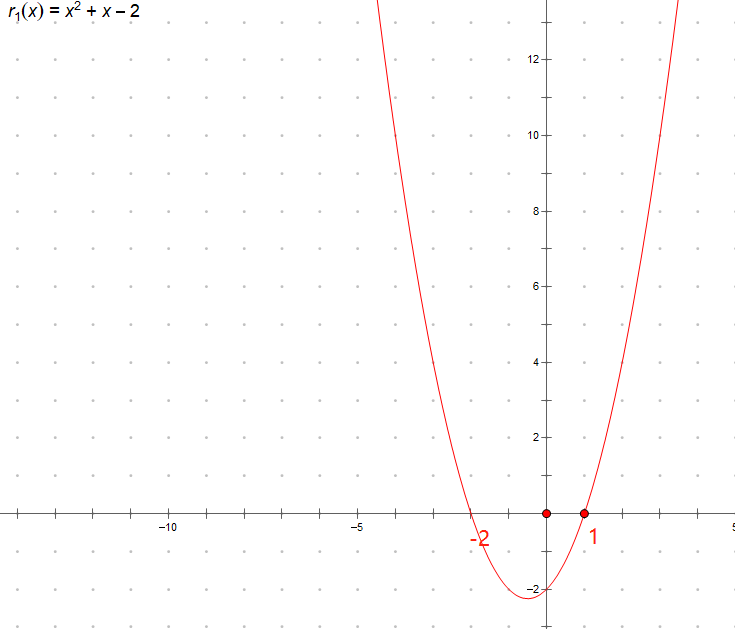05-05427711-061924
06-10534
01-31373
02-23305
08-1991
11-11286
06-264104
02-1627
07-291901
02-17353
11-0426
01-222840
11-11627
10-1381
06-0896